### My CPU

Homebrew CPU construction / házi készítésű processzor

### Feedek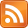## FunCPU - Architecture

### 2015.10.14. 21:45 Budapesti álmodozó

The picture below depicts the overall architecture of the FunCPU. It employs a 8 bit wide databus and a 9 bit wide address bus. Please recall, that although the databus is 8 bits wide, due to the nature of the tagged architecture only 7 out of 8 bits can be used externally (i.e. by the program), and one bit is reserved by the processor to interpret the data. So, from the programmer's point of view the CPU can be considered as a 7-bit CPU, which admittedly is a little odd.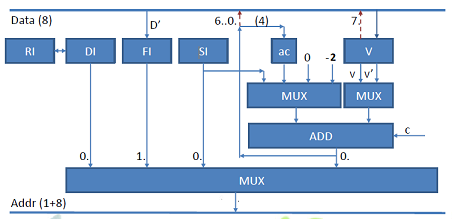More surprisingly, none of the familiar registers such as PC, SP (stack pointer), accumulator is available. Not even conventional status bits, such as Zero, Overflow flags, etc. can be located (note: although carry bit is present, but it cannot be referenced explicitly or even implicitly by the programmer. More on this later.). This is, because FunCPU employs a rather unconventional, delicate concept for program evaluation.

FunCPU supports 256 bytes of expression memory, in which expressions can be entered and reduced, and also has 256 bytes of function memory, which is dedicated solely to storing user-function definitions. The following internal registers are present:
- RI - reduction index register, is a 8 bit register used for function evaluation.
- DI - is the destination index register. This is a 8 bit register used to access and write destination symbols.
- FI - is the function index register. This is also a 8 bit register, which content is fetched upon entering a function. This register is being incremented as the function definition is being unfolded.
- SI - is the source index register. This is also a 8 bit register pointing to the current symbol of the expression to be reduced.
- ac - is the argument counter. This is a 4 bit register used to support function embedding.
- V - is the value register. This 8 bit register is somewhat analogous to the accumulator utilized in conventional processors, but again its value cannot be read or written by the programmer. V register is used as a termporal storage or a gateway between source and destination expressions.

Please notice that all registers are internal, none of them is exposed to the programmer. The programmer and the user program cannot read, refer to or manipulate these registers explicitly.

The address multiplexer selects among four possible address sources as follows:
- DI is selected (usually) when destination expression is extended/written.
- FI is selected when function definition is accessed.
- SI is selected when source expression is read.
- finally, the ALU output is selected (in a combination when SI and V' are added) to perform argument binding.

The right ALU MUX generates either V or V'. The left ALU MUX either generates a constant of the range from -2 to 1 (also depending on carry bit) or selects SI or ac as one of its inputs.

Please refer to ALU physical implementation for more details.

## FunCPU - Arithmetic Logic Unit

### 2015.10.11. 08:54 Budapesti álmodozó

The ALU module is very simple and straightforward. It is mounted together with the control board encoding logic on a single board. It performs a simple addition operation on the two operands as described in the following table. The two selector bits define the two input sources.

 ALU selector Input A Input B Carry Result Usage 00 0000 0000 (0) V 0 V Identity / copy 01 AC V’ 0 AC+V’ Argument counting   Only four bit value is used 10 1111 1110 (-2) V 0 V-2 Used to set AC to 1 argument, provided that V is zero beforehand 11 SI V’ 0 SI+V’ Argument binding 00 0000 0000 (0) V 1 V+1 Increment 01 AC V’ 1 *AC+V’+1 10 1111 1110 (-2) V 1 V-1 Decrement 11 SI V’ 1 *SI+V’+1

*these combinations are actually not used.
where V’ denotes 1111 11 V(1) V(0), where V(1) and V(0) are the two least significant bits of V.

Notice that the ALU is able to generate V (identity), V-1 (decrement), V+1 (increment) and V-2 based on the V value.

Please also observe that since this is a 7-bit CPU, it is not desirable to go out of the seven bit range. In other words, (without the tag interpretation) when V is holding a constant it cannot go beyond the range of 0..127 no matter if it is incremented or decremented. Therefore when storing the modified V value (here we mean solely V-1, V+1, but not V’) to the expression cell as defined by the destination register, the original most significant bit of the V register is fed back along with the possibly modified 7 bit part of the ALU result. This way the highest bit is always reserved and the actual constant part of the result value must lie in the 7 bit range.

Partially, in line with the aforementioned, the content of V register is not directly connected to the databus, instead, the ALU output (except for the MSB, which is coming from the V register) is released to the databus.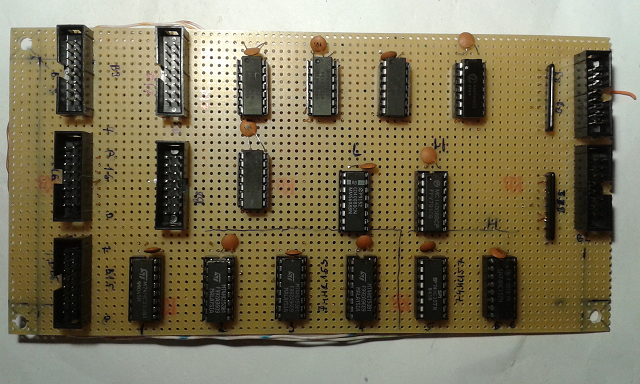Input sources are mapped by six multiplexers. Four 74HC153s are utilized to select among four sources (SI, AC, 0, -2), and two 74HC157s are used to select between V and V'. The outputs of these multiplexers are fed to two 4008s to perform the 8 bit addition.

Bus 15 connects register and ALU modules transferring the following signals to the ALU:

 V7 V6 V5 V4 V3 V2 V1 V0 SI7 SI6 SI5 SI4 SI3 SI2 SI1 SI1

Bus 16 supplies the full 8 bit ALU result to the register module. This value is used in the course of argument binding.

 R7 R6 R5 R4 R3 R2 R1 R0

Bus 9 has similar content, with a different arragnement. It transfers signal from the ALU to the RAM module.

 R6 R4 R2 R0 V7 R5 R3 R1

Bus 17 provides the signals to the ALU coming from the register modules as depicted in the table below.

 ALU_A ALU_B AC3 AC2 AC1 AC0 C PWR GND

Other buses are dedicated to signals related to keyboard processing. These are as follows:

Bus 8 is feeding RAM module with the encoded 8 bit data as defined by the user interface on the control board.

 D6 D4 D2 D0 PWR GND D7 D5 D3 D1

Bus 2 is a two way bus, accepting values from the control board (D0..D7) and also telling the control board what datalines can be active in a particular setup (i.e. when hotkeys are pressed) (DE0, DE1, DE6, DE7).

 DE6 D6 D4 D2 D0 DE0 PWR GND DE7 D7 D5 D3 D1 DE1

Finally, bus 3 accepts hot key signals from control board. Based on this information along with the 8 bit data determined by the 8 toggle switches (coming via the bus 2), the actual 8 bit data is generated, and then  transferred in bus 8 to the RAM.

 ZERO EOX ARG INC IF DEC PWR GND

## FunCPU - Test Module

### 2015.09.04. 22:24 Budapesti álmodozó

Below a simple tester module is depicted. It is designed to facilitate the testing of other modules.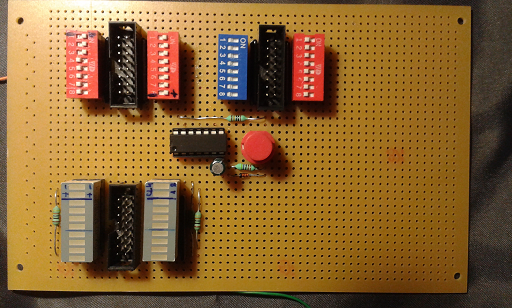It has one incoming databus of 16 bit width. Each of the lower eight Leds of the two ten segment Led bars corresponds to one bit in the databus. The upper two Leds of the led display on the left hand side, are as follows:
- The uppermost Led should be on, whenever the most significant bit of the left outcoming databus is connected to the ground.
- The Led below is on to denote that the least significant bit of the same outcoming databus is connected to the power supply.

The two uppermost bits of the Led bar on the right hand side indicates whether we are in clock phase 1 or 2.

The content of the outcoming databus on the left hand side can be manipulated with the two red DIP switches. This bus may also be used to provide power supply to other modules linked to the tester. In such case, the switches designated with the plus and minus signs should be set to their respective position. Recall, this can be checked by looking at the status Leds of the left hand side Led bar.

The data on the other bus on the right hand side can also be given with the 2 Dip switches (one blue and one red). There are however, two special bits on this bus. Bit 10 and 16 represents Clock 1 and Clock 2 respectively. All the other bits can be used and set arbitrary.

The red button next to the 74Hc14 Schmitt trigger is used to move from clock phase 1 (i.e. Clock 1) to clock phase 2 (i.e. Clock 2).

I hope that with this very simple module I will be able to test - among others - the register module, which is planned to be implemented next.

## FunCPU - Clock Generator

### 2015.08.03. 11:00 Budapesti álmodozó

Construction of FunCPU has begun with the clock generator. The module is already fully functional on its own, but space is left for incorporating more components for the EPROM chips storing the micro-program and for some control logic.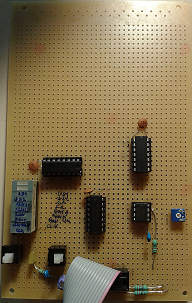An 555 is used to generate the base for the clock signals. There are two options to manipulate the clock frequency. A trimmer potentiometer is used to fine tune the frequency within two ranges selected by the right switch. In one position the base clock frequency ranges roughly from 1.4 Hz to 64 Hz, which can be used for debugging, in the other position this covers the interval from 14.5 Khz to 47 Khz, which is suitable for normal operations.

A 74HC74 is used to ensure the following:
whenever the two-phase clock starts:
- it starts with phase 1
- it starts with full cycle
- signals when two-phase clock starts
furthermore the duty cycle is guaranteed to be roughly 50%.

A 74HC161 fed by the two-phase clock is applied to generate micro-sequence (from 0 to 3).
A 74HC02 is used as an RS flip-flop to debounce the Edit/Run toggle switch.and also to enable/disable clock signals.

The LED-bar is used for debugging and can be turned on/off by the left switch.

 not used not used US0 least significant bit of the micro-sequencer US1 most significant bit of the micro-sequencer CLK2 phase 2 of the master clock CLK1 phase 1 of the master clock RUN bright, if in Run mode EDIT bright, if in Edit mode CLK RUN to indicate whether clock is enabled CLK Master clock with half of the frequency of the base clock

The following BUS  ("B6") is impelemted on the board:

 RUN_OUT (15) EDIT_OUT CLK2 not used CLK1 not used CLK_RUN(3) VCC (1) GND (16) US0 US1 not used not used RUN_IN EDIT_IN(4) not used(2)

RUN_IN and EDIT_IN are the incoming signals (with prell) representing Run or Edit respectively. Whereas RUN_OUT and EDIT_OUT are the debounced signals. The rest of the signals are described above along with LED indicators.

Finally, we can see the clock generator in action. Before the clicking noise, FunCPU is in "Edit" mode, therefore clock is basically idling. When Run/Edit toggle is switched to "Run" position, then at the next clock cycle the clock generator starts to operate as indicated by the the blinking LEDs.

## Control Panel

### 2014.10.08. 21:36 Budapesti álmodozó

Ok, here is a photo of the real control panel. It is a little bit different from the original design. It does not look that  spectacular, but has decent functionality.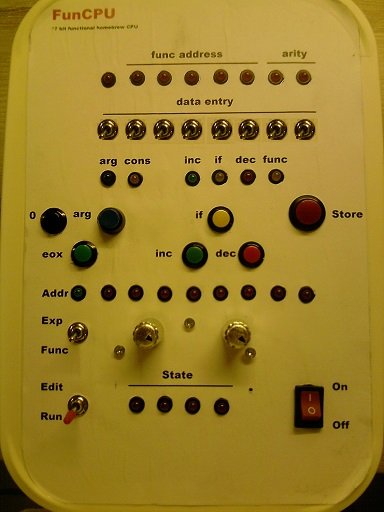The upper section includes 8 LEDs and 8 toggle switches. These are used for data entry as indicated by the label in between. In addition to showing the full 8-bit value in the memory, this value is decoded and its type (remember, we are dealing with a tagged architecture) is indicated below by the type indicator LEDs.

If the data is an argument, then the blue “arg” LED will be active, if a constant is found, then the “cons” LED is bright and so on. So by looking at these LEDs one immediately will know the function of the data being observed.

Also six function shortcut buttons are introduced to facilitate the data-entry. One may have a choice of selecting the data with the 8 toggle switches or using one of the function buttons, or alternatively having a combination of them.

“0” is used to store zero value (also interpreted as True). This comes handy, since one does not have to bother about turning the toggle switches to zero position to be able to enter 0. “eox” (indicating end of expression) does the opposite, it gives a \$FF value. “arg” button can be used along with the two least significant toggle switches to enter argument number. The use of “inc”, “dec” and “if” should be self-explanatory.

I expect that the majority of functions and expressions can be simply entered using the functional buttons, and toggle switches will be rarely used.  On one hand this would speed up data-entry significantly, on the other hand function indicator LEDs will be major help in debugging.

Once the actual data is selected, the “Store” button must be pressed to store the data into the memory.

To where and from where this 8-bit is actually coming, is determined by the address control gizmos. The toggle switch “Exp/Func” switches between expression and function memory. Whereas the two silver knobs enables us to select a full 8-bit value (four bit each).

All the above buttons, switches, knobs work only in “Edit” mode, but do not have any effect in “Run” mode. To choose between the two modes, one has to toggle the “Edit/Run” switch.

Please note that the LEDs are working in the same fashion regardless of the operating mode, except, that in running mode the address is determined by the processor internal state, therefore the data being shown (both by the data and address LEDs) reflects the actual computational state. The actual 4-bit state is indeed displayed by the state LEDS.
0000 is the initial, starting state. 1111 is the final state for successful computation.

Finally, the big red button on the right bottom is used to turn the computer on or off.

## Boxing Day...

### 2014.09.18. 21:48 Budapesti álmodozó

It is high time I had gone to look for a suitable box to be the case of the FunCPU. I have decided on the one below. It is quite ugly and girlish actually, but it was the cheapest option.At least this will be the choice for CPU-case of my first attempt to build the CPU.

I expect to implement all functionality using 5 or 6 modules. Each module will be implemented as a single board. These boards should fit into this box.

## Overcoming Some Limitations

### 2014.08.19. 21:00 Budapesti álmodozó

The FunCPU (in its current version) supports only numerical computation, more precisely, operations with integers. Functional languages, such as Lisp, Clean, etc. come with a richer set of data types and also have some kind of type construct capability. For example, Lisp as its name suggests mainly operates with lists.

Moreover, the maximum number of arguments is limited to four. Four arguments are usually enough to demonstrate some simple, yet interesting mathematical functions, but more complex scenarios require usually more arguments.

Hereunder I will be sketching some workarounds to overcome the aforementioned limitations, but please beware that these will bear mainly theoretical relevance.

More Arguments

To increase the number of arguments supported, one can use any pairing function, which is a bijective function mapping two natural numbers to another. For instance, the Cantor pairing function is defined as follows:

P(x,y):=0.5 * (x+y) * (x+y+1) + y

Even more arguments can be encoded, if we use nested pairing functions, that is essentially use the Cantor tuple function. For more information please refer to:

Lists, Records

Similarly, lists (or record-like structures) can be encoded using the so-called Gödel’s encoding, so that, a list or string of n consecutive characters is encoded as follows:

enc(x1, x2, …, xn) := 2^x1 * 3^x2 * … * Pn^xn, where Pn denotes the nth prime.

Obviously, due to the 7-bit architecture such encodings are rather limited, but still theoretically feasible.

## Evaluating 1+1

### 2014.08.16. 22:00 Budapesti álmodozó

It is high time we turned our attention to a complex mathematical problem. Namely, how FunCPU computes:
1+1=?

Please recall that the definition of "add" is stored at 00 (referenced by 81) is as follows:

add(x,y):=   FD 7F 7E FC 81 7E FE 7F FF

The following is an extract from the FunCPU expression memory, which clearly indicates how a simple expression is stored, evaluated and reduced to a final result.

: 00 01 02 03 04 05 06 07 08 09 0A 0B 0C 0D 0E 0F
00: 81 01 01 FF FD 01 01 FC 81 01 FE 01 FF FC 81 01
10: 00 FF FC FD 01 00 FC 81 00 FE 01 FF FC FC 81 00
20: 00 FF FC FC FD 00 00 FC 81 00 FE 00 FF FC FC 00
30: FF FC 01 FF 02 FF .............................

Where different colors denote different expressions as illustrated below:
- Initial expression 1+1
- the result
- first instance of unfolded, bounded function definition
- third cyle, after "if" condition is evaluated

As the above extract reveals, in the first cycle, the function definition is unfolded with the arguments bounded simultaneously. Then the "if" expression is evaluated. Since second argument is not zero, the else part is copied to the third cycle. Note, that the second argument now is reduced, thus add(1,0) is called. The result of this call is incremented, thus effectively expression became 1+add(1,0).

The following extract depicts the state of the expression memory at the final stage, when fac(5) is calculated. Result is shown in green. One can see, that evaluating fac(5) has required quite a many cycles (this is basically due to the low level of efficiency of the built-in functions inc and dec), and the original expression calling fac(5) is overwritten.

00 01 02 03 04 05 06 07 08 09 0A 0B 0C 0D 0E 0F
00: FF FC FC FC 75 FF FC FC 76 FF FC 77 FF 78 FF FC
10: FC FD 03 71 FC 81 71 FE 03 FF FC FC FC FC FC 81
20: 71 02 FF FC FC FC FC FC FD 71 02 FC 81 02 FE 71
30: FF FC FC FC FC FC FC 81 02 70 FF FC FC FC FC FC
40: FC FD 02 70 FC 81 70 FE 02 FF FC FC FC FC FC FC
50: FC 81 70 01 FF FC FC FC FC FC FC FC FD 70 01 FC
60: 81 01 FE 70 FF FC FC FC FC FC FC FC FC 81 01 6F
70: FF FC FC FC FC FC FC FC FC FD 01 6F FC 81 6F FE
80: 01 FF FC FC FC FC FC FC FC FC FC 81 6F 00 FF FC
90: FC FC FC FC FC FC FC FC FD 6F 00 FC 81 00 FE 6F
A0: FF FC FC FC FC FC FC FC FC FC FC 81 00 6E FF FC
B0: FC FC FC FC FC FC FC FC FC FD 00 6E FC 81 6E FE
C0: 00 FF FC FC FC FC FC FC FC FC FC FC 6E FF FC FC
D0: FC FC FC FC FC FC FC 6F FF FC FC FC FC FC FC FC
E0: FC 70 FF FC FC FC FC FC FC FC 71 FF FC FC FC FC
F0: FC FC 72 FF FC FC FC FC FC 73 FF FC FC FC FC 74

## On (in)Efficiency of FunCPU

### 2014.08.11. 21:45 Budapesti álmodozó

On Data and Program Representation

The computation (expression reduction) process is based on string manipulations (copy, insert, replace, etc.). The input string is scanned symbol by symbol until the string is reduced to an integer. This may happen in some cases, but may not in others. In the course of this scanning/copying operations built-in functions as well user-functions are evaluated. We will refer to a series of such scan/copy activities as cycle, until the expression end tag is reached. Please note that there is some optimization at work, so that, for instance, when copying the string

FC FE FC 00 (representing inc dec inc 00)

it is immediately reduced (that is to say in the very same cycle during scan/copy loop) to

FC 00 (representing inc 00), and then to 01

In this way some cycles can be saved. Otherwise the chain of reduction would be like this:

FC FE FC 00 => FC FE 01 => FC 00 => 01

Note each reduction happens in a separate cycle.

Still the clear disadvantage of this evaluation process lies in the facts that:

• high number of cycles (this is further detailed in the section below) are required;
• a relatively big number of symbols are copied/moved in each cycle.

On CPU Cycles

The FunCPU is quite inefficient, that is to say, each calculation requires quite many cycles. Actually too many. This is basically due to the very low level built-in functions, i.e. inc and dec. To illustrate this, just imagine how two integers are added. For example, x+y requires just y cycles to increment x (using the definition of “add” function as outlined below), that is, to add y to x, not to mention the additional cycles required for unfolding the “add” function, evaluating the if-then-else expression, etc.

add(x,y):=  if  y=0  then  x  else  inc(add(x,dec(y)))

It is worth noting that although addition is commutative, the computation of x+y and y+x requires different number of cycles (and thus execution time) provided that x and y are different integers. Of course, to eliminate this undesired property, one can define a more clever addition function, which swaps its arguments initially in order to minimize the number of cycles required.

It is quite straightforward to think of introducing higher level functions, such as addition, subtraction, multiplication, division, etc. Needless to say, that with such richer set of built-in function the efficiency of computation process is highly increased at the price of a more complex architecture.

## Some Functions and Predicates

### 2014.08.07. 21:48 Budapesti álmodozó

Let us have a look at some elementary functions and predicates to have a better look and feel how FunCPU works. The definitions of these objects will be suppmented with their assembly code counterparts written directly in FunCPU machine code.

In the subsequent section, the following encoding is used:

FC - increment function
FD - if-then-else
FE - decrement function
FF - end of expression

Identity function

I(x):= x

7F FF

Constant function

C(x):= c

„c” FF, where c=00..7B

Please note that as 7C and above, up to 7F denote arguments, therefore it is impossible to write a constant function directly, which returns these values. For example, as we have seen 7F FF denotes the one-argument identity function.

Fortunately we can overcome this limitation by defining the function "7C FF" as follows:
FC 7B FF

That is, essentially, incrementing the value 7B to get the 7C.

Or analogously,

FE 00 FF

represent the constant function 7F.

Please note this will work, as functions will be evaluated by unfolding the function definition and inserting into the expression memory. In the expression memory no distinction is made between constants and arguments, they are all being treated in the same way.

Few Predicates

not(x):=  if  x  then  1
else  0

FD 7F 01 00 FF
=(x,y):=  if  y=0  then  x else  =(dec(x),dec(y))

FD 7F 7E 81 FE 7E FE 7F FF

Assuming that "=" definition is stored at address 00.

>(x,y):=  if  x=0  then  not(y) else  >(dec(x),dec(y))

FD 7E 88 7E 91 FE 7E FE 7F FF

Assuming further, that "not" and ">" definitions are stored at 10 and 20 respectively.

Some functions

add(x,y):=  if  y=0  then  x  else  inc(add(x,dec(y)))

FD 7F 7E FC 81 7E FE 7F FF

Assuming that "add" is stored at 00.

mul(x,y):=  if  y=0  then  0   else add(x,mul(x,dec(y)))

FD 7F 00 81 7E 91 7E FE 7F FF

Assuming that "mul" is stored at 20.

fac(n):=    if  n=0  then  1  else  mul(n,fac(dec(n)))

FD 7F 01 81 7F 98 FE 7F

Assuming that "fac" is stored at 30.

As one can see, a whole set of interesting and useful functions and predicates can be built, one based on another. So the three atomic functions along with the potential of user defined functions and (recursive) function calls make it possible to define arbitrary complex functions (in theory, in reality memory constraints put limitation on the set of definable functions).

A more complex function is the Ackermann function, which is recursive, but not primitive recursive.

ack(m,n):=
if m=0 then inc(n)
elsif n=0 then ack(dec(m),1)
else ack(dec(m),ack(m,dec(n)))

FD 7E FC 7F FD 7F 81 FE 7E 01 81 FE 7E 81 7E FE 7F FF

where "ack" is defined at location 00.

We can already "feel" that the FunCPU is Turing-complete...

## Intefacing with the Functional CPU

### 2014.07.29. 22:32 Budapesti álmodozó

Now let us take a look at how the functional CPU (FunCPU for short) can be programmed in reality, from the programmer’s point of view.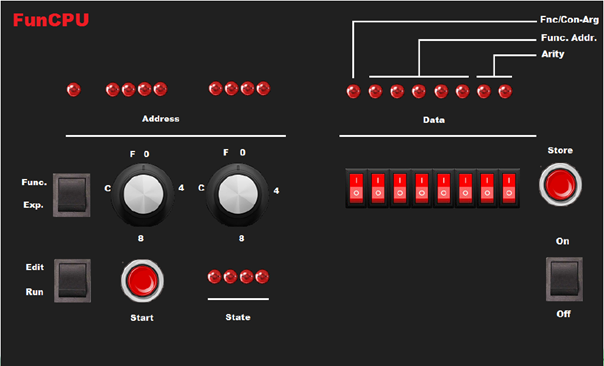This will be covered by looking at each gizmo  (i.e. button, switch, LED, etc.) on the front/control panel.

On the bottom right side, the purpose of the power switch is self explanatory.

On the  bottom left side,  the switch is used to select between run and edit mode. In edit mode, the functions and/or expressions can be entered/modified/displayed. The computation can be initiated by pressing start button, only when the edit-run switch is turned to run mode.

The third switch is provided to switch between editing functions and expressions.

The two rotary switches next to it, are used to enter the 8-bit physical address in hexadecimal mode. The current physical address (both in edit or run modes) are indicated by the 9  address indicator LEDs. The most significant, the 9th bit LED is on, when expressions are edited in edit mode, or when in run mode the expression symbol is selected by the CPU.

Similarly, the current data byte stored at the actual address is displayed by the LEDs labelled Data.  Under these LEDs the eight switches are provided to enter or modify data in binary mode.

Store button (only working in edit mode) is actually used to enter the 8 bit value in either to function memory or expression memory depending on the position of the Func/Exp switch. When the store button is released, the previous data byte displayed by the 8 data LEDs, is overwritten, and the new value is displayed.

The most significant bit (8) denotes whether the value is a function (LED is on) or a constant (or possibly an argument) (LED is off). The following 5 LEDs as suggested by their corresponding label give the physical address of the user-function. The actual address can be obtained by multiplying this 5 bit value by 8 (or simply shifting it left three times). The last two LEDs encode the arity of a user-defined function: 00 , 01, 10, 11 represent arity 4, 3, 2 and 1 respectively.

Finally, some important values in binary form:

• 0000 0000 – zero
• 0111 11xx – argument 1, 2, 3  or 4 (in function mode)
• 1111 1100 – dec function
• 1111 1101 – if function
• 1111 1110 – inc function
• 1xxx xxaa – user-defined function at address %xxxx x000 with arity aa, provided that it is different from the code of the 3 built-in functions described above
• 1111 1111 – end of expression / function

Current machine state is indicated by the four LEDs labelled as State. The initial, starting state is 0000, whereas 1111 denotes the succesfull termination of the computation. In the latter case, the result of the computation is displayed by the data LEDs in binary model.

## FunCPU - Passing Arguments

### 2014.07.23. 20:13 Budapesti álmodozó

It is not trivial how to handle argument passing in the lack of stack, or any dedicated storage for parameters. Consider the following examples:

fac (n):= n*fac(n-1)

fac(add(2,3))?

ack(add(fac(fac(2)+fac(1)),fac(1)),1)?

I wanted a simple solution, which still works without posing serious restrictions. I wanted arbitrary function calls with arbitrary depths.

I have come up with a very simple solution. The evaluation of a function is deferred up to the point when all of its parameters become constant. Referring the the term fac(add(2,3)), first add(2,3) is reduced to 5. Only after that is the fac function reduced by applying the previous result as an argument, that is, fac(5), which in turn is reduced to 120.

In similar fashion the evaluation of the second example happens.

First, fac(2), fac(1) (this appears twice) are reduced. Please note that there is a lot of potential to exploit parallelism and for optimization. These expressions can be reduced in paralllel, furthermore fac(1) should be calculated only once.

In the second step fac(fac(2)+fac(1)) is evaluated. Note: this time we have the constant value both for fac(2) and fac(1). In the third step add is performed, finally ack is executed.

## FunCPU - Parenthesis, Precedence

### 2014.07.22. 17:03 Budapesti álmodozó

When evaluating expressions, the CPU must exactly know how to evaluate an expression, what function/expression parts should be evaluated first, next, and last etc. Therefore the representation of expressions and functions must be unambigous with respect to parenthesis and priorities.

In mathematics, by convention some functions take precedence over other functions (e.g. multiplication has higher precedence than addition). To override such precedence, parenthesis can be used. See, for instance:

4+5*6 = 34
(4+5)*6=45

It is natural to think of introducing then parenthesis to make the meaning of expressions exact. However, this could result in additional complexity for evaluation, not to mention the fact, that parenthesis must be encoded by extra symbols.

Something simple was required, which could be implemented with the minimum hardware. So I have decided on using the Polish prefix notation. The above two expressions thus become the following respectively:
+ 4 * 5 6 = 34
* 6 + 4 5 = 45

As long as the arity of functions are fixed and known in advance, this notation represents the expressions unambiguously.

To implement this in hardware a simple 4 bit counter is used. The scope of a function then can be determined by using this counter as follows:

• Counter initial value will be the arity of the function of which scope is to be determined.
• If a constant is found, then counter is decreased by one.
• If a function with arity n is found, then the counter is increased by n-1.
• If counter reaches zero, then this means it was the last symbol in the function scope.

The following example demonstrates the use of the counter to determine the scope of the first „fac":

if 2 fac + fac 2 fac 1 if ... ac=1
if 2 fac + fac 2 fac 1 if ... ac=2 =1-1+2
if 2 fac + fac 2 fac 1 if ... ac=2=2-1+1
if 2 fac + fac 2 fac 1 if ... ac=1 =2-1
if 2 fac + fac 2 fac 1 if ... ac=1=2-1+1
if 2 fac + fac 2 fac 1 if ... ac=0=1-1

Please note that actually this is achieved in hardware a little bit differently. As arity is encoded in two’s complement, counter is increased by one, if a constant is found, and it is decreased when a function in scope is encountered. Also its initial value stores the function arity in two’s complement.

The choice of a 4 bit counter seems to be rather limited, but it is in balance with other hardware figures (memory, registers, etc.). Given a function f with 4 arguments, due to the 4 bit limitation the term
f(f(f(f(... is still fine (counter=4+3+3+3=13), but
f(f(f(f(f(... cannot be evaluated (counter=4+3+3+3+3=16), however,
f(f(f(f(const,f(... is ok again (4+3+3+3-1+3=15).

## FunCPU - Evaluation Strategy

### 2014.07.22. 16:52 Budapesti álmodozó

It is not trivial how expressions should be reduced, i.e. evaluated. I have already mentioned that while some strategy could be fruitful in reducing an expression in a given interpretation, others may be not.

Let us have a look at the following simple example of evaluation of the factorial function is defined under the alias fac.
fac(n):= if n=0 then 1 else n*fac(n-1)

The reduction of fac(1) may lead to

if 1=0 then 0 else 1*fac(0)
If 1=0 then 0 else 1*(if 0=0 then 1 else 0*fac(-1))
If 1=0 then 0 else 1*(if 0=0 then 1 else 0*(if -1=0 then 0 else -1*fac(-1))) .......

We can easily see that the reduction goes on forever, it never terminates.

The goals of the evaluation strategy are as follows:

• Must terminate (simple applicative rules may result in infinite loop)
• Must be efficient (as possible).
• Must be simple (suitable to be represented in hardware directly).

The following strategy will be applied:

A user-defined function is reduced if and only if all of its arguments are constants. Similarly, inc and dec are reduced only, if their arguments are literals. As far as if-then-else is concerned: if its condition part is constant 0 (i.e. true), then it is reduced to exp1. If the condition part is a constant other than 0, then it is reduced to exp2. Othewise the reduction is postponed.

Corollary: all parameters passed to functions are constants.

The reduction algorithm is as follows:

1. Scan input expression symbol by symbol.
2. Copy symbol from source to output if symbol is not a function, or its a function but cannot be reduced in its current form (i.e. contains at least one argument, which is not a constant).
3. Evaluate functions (if, inc, dec, and user-defined) if possible. That is, rewrite the function call by replacing the call with the function definition with bounded arguments.
4. If EOX symbol is found, then the whole cycle restarts. Note: in the next cycle: the current output becomes the input and vice versa.
5. Stop, if the first symbol is a constant. This literal is the result of the reduction.

It is clear that inc, dec terminates immediately, thus reduces the length of expression. The expression size also shrinks when in the expression „if cond exp1 exp2” cond is a constant. Eventually everything is based on/can be reduced to the three built-in functions. Therefore sooner or later, all „if”, „inc”, „dec” are evaluated/reduced, thus the evaluation terminates (if this is possible).

## FunCPU - Memory Models

### 2014.07.21. 14:05 Budapesti álmodozó

Needless to say that not only initial and final expression, but also temporary expressions should be stored in memory somehow. The way of how expressions are represented has impact on hardware design.

During reduction the cycles, expression symbols are being copied one by one. When a function symbol is found, then the symbol itself along with possible other consecutive symbols are rewritten in accordance with the function definition (this is used in a broader sense, and includes definition of built-in functions).

Please note that the following discussion is related only to expression evaluation, as user-defined functions are stored in a separate memory, possible in ROM.

Separate source and destination memory

Separate fragment of memory is used for source and destination expressions. Upon each reduction cycle the role of the memories are swapped. Source memory will become destination and vice versa. This is illustrated in the figure below.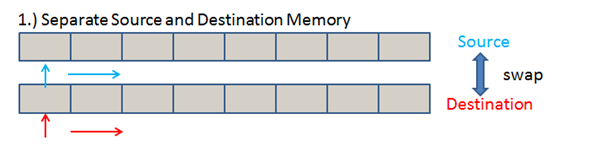If l is the longest possible size (in memory cells including EOX) of the expression during the evaluation, then the minimum memory requirement is 2xl cells.

Shared memory, with index increasing and decreasing

A shared memory can also be used. In this case source index is increasing, whereas destination is decreasing while copying symbols. After each evaluation cycle, the role of the indexes will be swapped again. This memory model may be more econimic, since in some cases it will be suitable to reduce expressions over half of the memory size, provided that the reduced expression becomes smaller, satisfying the following equation, which in fact should hold in every cycle: e+r≤m, where e, r and m denote expression size (excluding EOX), reduced expression size and memory size respectively.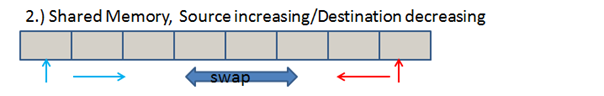Shared memory with index following

The last model is also comparable to the previous one in econimical terms, as the same equation among expression lengths and memory size must hold. However, it has the additional benefit, namely that index roles do not need to be changed. This simplifies hardware design, as multiplexers (swapping the source and destination registers) and its relevant control signal and logic can be avoided.  As source index reaches the end of input expression marked by the EOX symbol, it should just copy and step over that symbol and the continue with the next reduction cycle. If memory end is reached, then indexes will wrap around starting from a lower location.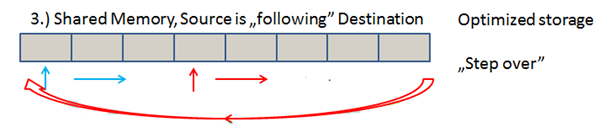## FunCPU - Function Encoding Schema

### 2014.07.18. 22:43 Budapesti álmodozó

In the previous post we have seen how 8 bit symbols represent literals, arguments, functions, etc. Similarly, it was vital to have a good and efficient function encoding. Basically in the context of function encoding we need to be able to answer the following question:

• Where does the function definition begin?
• Where does it end?
• How many arguments does a function have?

First, I have planned to use function identifiers as references to physical memory locations. So, for instance, functions will be indexed by starting from 0. But this indirection could cost a lot in terms of hardware.

Instead, I am using the actual symbol to determine the function address directly. The most significant bit itself signals whether the symbol in question is a function (it is a function, if it is on, except for EOX). The consecutive 5 bits multiplied by 8 will actually give the function physical address. For example, %1aaa.aaxx - function definition will start at physical binary address %aaaa.a000.

The observant reader may be wondering what the two least significant bits are used for. They represent the argument count encoded in two-complenent form as follows:

• %00 - 4 arguments
• %01 - 3 arguments
• %10 - 2 arguments
• %11 - 1 argument.

Again, this rather strange encoding is selected to facilitate the processing in hardware.

In the light of the aforementioned, a full function encoding works as follows:

• %10000000 - is a user-defined function with 4 arguments, of which definition starts at address \$00.
• %10000111 - is a user-defined function with one argument with definition beginning at address \$08.
• %11111010 - is a user-defined function with 2 arguments definition at address \$F0.

Please also note that built-in function "if" is encoded in accordance with the above arity encoding, i.e \$FD (symbol encoding "if") represents a 3 argument function. I should mention that the encoding of inc/dec does not conform to this, but they are being treated differently as we will see.

## FunCPU - Tagged Architecture

### 2014.07.16. 22:03 Budapesti álmodozó

In the memory, different type of symbols are stored together. It is essential for the CPU to be able to differentiate among them. Therefore type information is required to be assigned to each unit of information. This piece of information is tagged to each symbol. A lot of effort has been dedicated to come up with an optimal encoding. I wanted to have an encoding/tagging scheme, which was very economical and yet efficient.

I have decided to use a single 8 bit RAM/ROM for memory, so everything (including the actual data and its type information) must fit there. With 8 bits of data I could encode the following information:

• 7 bit literals
• built-in functions
• user-defined functions
• expression terminator.

The latter is used to mark the end of an expression (essentially the core of the functional program) or a function.

I have further classified the 8 bit symbols with respect to the above categories as follows:

• zero constant, which also represents True;
• other constants
• arguments;
• inc function
• dec function
• if function
• user-dedinable function
• expression terminatior (EOX).

This classification, as we will see, will heavily facilitate the processing of the expressions.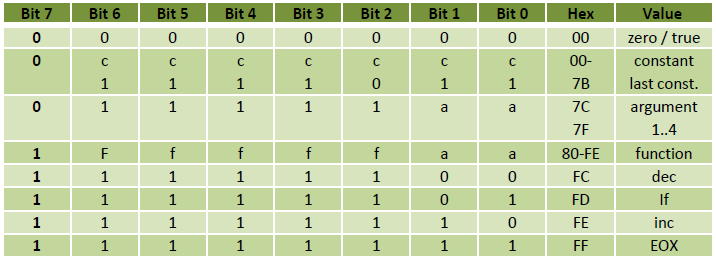## FunCPU - Computational Model

### 2014.07.13. 22:40 Budapesti álmodozó

As the computational model will be purely functional without any imperative elements (which could introduce side effects), a simple expression rewriting system will be adequate.

User-defined functions will make up a library, which will in turn, also define the rewriting rules. Functions from library may refer to each other (i.e. one function can be used in another).

The expressions to be evaluated can be made of:

• built-in functions;
• user-defined functions (as stored in the library);
• literals.

EOX symbol will mark the end of such exrpession. Program execution will be not other, than evaluation of a closed expression (it is called such, because it may not contain any variables or quantifier) following the rewriting rules (as defined in the library) transforming an expression into a chain of expressions, and finally into a constant.

If it is possible to reduce the initial expression into constant in finite steps, then the evaluation stops succesfully, and we say that the expression is defined. Otherwise we say that the expression is undefined. The latter may happen

• if expression or function deifinitions are not well formed, that is, they do not obey syntactical rules (e.g. a function is called with less or more arguments, than as its definition states,etc.);
• if an infinite loop is encountered (via recursion);
• if the particular evaluation strategy causes non-termination. This implies that there may co-exist other evaluation strategies, such as that provided that they are followed, the same expression with the same library (also called interpretation) can be reduced succesfully.

For example:

Library:
f(x,y) :=x+y
g(x,y,z) :=f(x,y)-f(y,z)

Closed expression
g(3,2,1)+f(2,2)

Execution:
g(3,2,1)+f(2,2) = ( f(3,2) – f(2,1) ) + (2+2) =( (3+2) – (2+1) )+ (2+2) = 6

Please note that as a function may refer to itself, it enables recursive function definition, and hence recursion. Along with function composition and atomic functions (inc, dec, if) it gives a real computation power to this simple model.

## FunCPU - Instruction set

### 2014.07.10. 21:42 Budapesti álmodozó

FunCPU will operate with 7-bit numerals (to understand why see the next couple of posts) and will directly support the following built-in functions:

inc(x) is used for incrementing a value, that is for any x: x+1=inc(x).

dec(x) is used for decrementing a value in the similar fashion.

if-then-else is used for conditional evaluation. if cond then exp1 else exp2 will yield exp1 if and only if cond is true, otherwise it will yield exp2 (assuming that evaluation of the condition terminates).

In addition to the above built-in functions, user definable functions may be entered arbitrary in the available 32 slots. These functions may use the built-in functions as well as any other user-defined functions. Functions can be embedded one into other at arbitrary depth. Functions can be created with arity 1, 2, 3 and 4. Constant functions still can be constructed, but they must have at least one argument. Thus, for example, if F(x) is a one-argument constant function, then F(x)=c for any x, where c is a constant.

Functions will only accept numeral constants as arguments (no higher order functions are supported), and will return also numerical value. Note: „0” represents True, any other value is considered to be False by convention.

By design, no advanced data structures (i.e. list, record, object, etc. ) will be supported natively.

At first it may seem suprising that with this very limited instruction set (and of course with the poweful construct of function embedding), any computable function can be defined and thus computed within this model, provided that its encoded algorithm (and also all expressions as a result of the function evaluation) fits in the memory.

## FunCPU - Goals

### 2014.07.09. 14:28 Budapesti álmodozó

It has been a long time since my last CPU project, which focused on a very simple 8 bit CPU. Nothing groundbreaking, just a very simple variation of an accumulator machine.

This time I have set out a different, more ambitious goal. I have wanted to create still something simple, but original unconventional architecture, which is still useful.

I had considered to target a CPU optimized for logical programming, but finally I have decided on a CPU with an instruction set and architecture, which natively supports functional programming.

A tagged architecture is selected. Basically, this means that each unit of information carries its own type information. A special instruction set will be carefully selected, which would be most useful to support creation of functional programs in assembly or even in machine code. The whole design will be very simple, yet powerful enough to perform arbitrary complex computations (in theory) to be Turing-complete.

It will have

• no PC;
• no SP or stack;
• no conventional user readable flags;
• no user readable registers (not even an accumulator in the conventional sense);
• no branching/jumping instructions;
• no I/O operations

So, what is what we have?

• 7 bit literals (yep, not mispelt!);
• 3 built-in,
• 32 user-definable functions;
• ROM – to store functions (256 bytes);
• RAM – to evaluate expressions (256 bytes);
• 8 bit data bus;
• 8/9 bit address bus.

### Címkék: cpuhomebrewfunctional programmingunconventionalTuring-complete